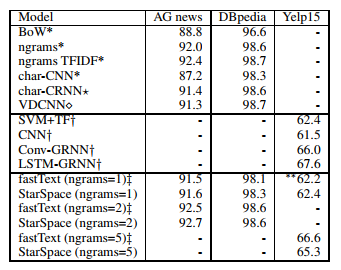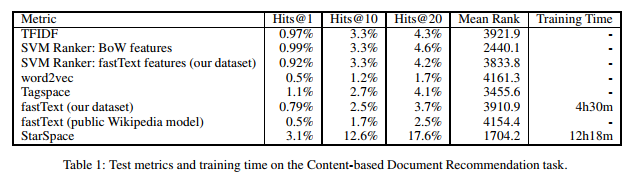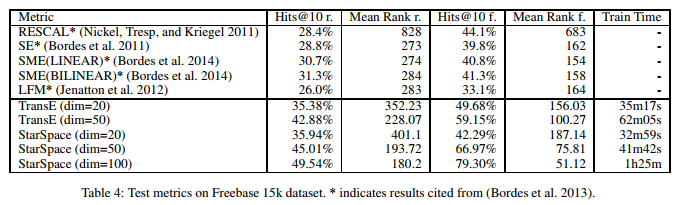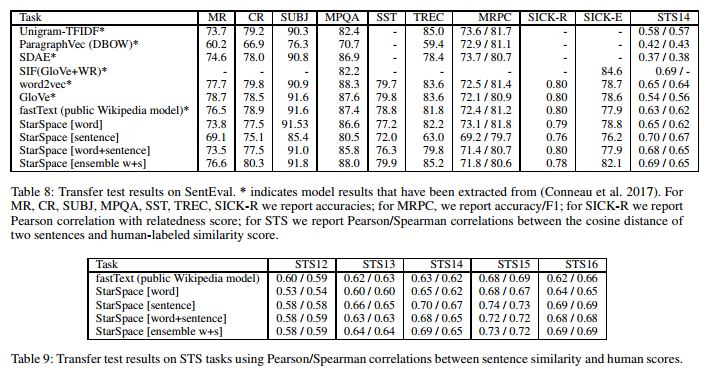# StarSpace: Embed All The Things! (Paper Summary)

Here is the link to the paper. C++ code for this available here.

The main contributions are:

• An embedding learning algorithm that generalizes across diverse problems.
• Embeddings of different types can be compared with each other. For example, a user entity can be compared with an item entity in the recommendation problem.

## Model Details

The StarSpace model consists of learning entities. Each entity is described by a set of discrete features (Note that the model does not generalize to continous features). The goal is to learn the $$D \times d$$ matrix, where $$D$$ is the number of features and $$d$$ is the length of the embedding vector. An entity $$a$$ is represented as $$\sum_{i \in a}{F_i}$$, where $$F_i$$ is the $$i^{th}$$ $$d$$-dimensional feature (row) in the embedding matrix.

The following loss is minimized during the training:

$\sum_{(a,b)\in E^+\\b^- \in E^-}{L^{batch}(sim(a,b), sim(a,b_1^-),...,sim(a,b_k^-))}$

The set of positive entity pairs $$E^+$$ and the set of negative entity pairs $$E^-$$ are problem specific. $$k$$-negative sampling strategy (same as the word2vec paper) is used to sample the negative entities $$b_i^-$$. Similarity function $$sim(\cdot,\cdot)$$ is either cosine similarity or inner product. And the loss function $$L_{batch}$$ is either ranking loss or negative log loss of softmax.

## Experiments

• Multiclass classification: $$E^+$$ and $$E^-$$ simply come from the text classification dataset. They test StarSpace model on 3 different datasets:
• AG news: It’s a 4-class text classification task given title and description fields. Contains 120k training examples, 7600 test examples, 4 classes, ~100k words and 5M tokens.
• DBpedia: Wikipedia article classification problem. Contains 560k training examples, 70k test examples, 14 classes, ~800k words and 32M tokens.
• Yelp reviews dataset from 2015 Yelp Dataset Challenge: The problem is to predict the full number of stars given the review texts. 1.2M training examples, 157k test examples, 5 classes, ~500k words and 193M tokens.• Content-based Recommendation: This task involves recommending social media posts to users based on the history of their likes. A post can be represented as a bag-of-words. The dataset consists of 641385 users and 3119909 articles. The problem is to predict the $$n^th$$ article given the last $$(n-1)$$ articles.The metrics for evaluation include [email protected], i.e. the proportion of correct entities ranked in the top k for $$k$$ = 1, 10, 20 and the mean predicted rank of the clicked article among the 10,000 articles.
• Multi-Relational Knowledge Graphs (Link Prediction) Freebase 15k dataset consists of a collection of triplets (head, relation_type, tail). (Obama, born-in, Hawaii) is one such example. The task is to predict either the head in (?, relation_type, tail) or tail in (head, relation_type, ?). The dataset consists of 14951 concepts and 1345 relation types. There are 483142 triplets in the training set, 50000 in the validation set and 59071 in the test set.The evaluation metrics include the mean of the predicted ranks (i.e. the rank of the head and the rank of the tail) and the [email protected]
• Information Retrieval and Document Embeddings
• Learning Sentence Embeddings: They use the SentEval tool from facebook research to compare the effectiveness of sentence embedding models on 14 transfer tasks including binary classification, multi-class classification, entailment, paraphrase detection, semantic relatedness and semantic textual similarity.